• 最小生成树Prim算法算法简介图解 算法简介 普里姆算法（Prim算法），图论中的一种算法，可在加权连通图里搜索最小生成树。意即由此算法搜索到的边子集所构成的树中，不但包括了连通图里的所有顶点，且其所有边的权值...

算法简介

普里姆算法（Prim算法），图论中的一种算法，可在加权连通图里搜索最小生成树。意即由此算法搜索到的边子集所构成的树中，不但包括了连通图里的所有顶点，且其所有边的权值之和亦为最小。

图解

1、原始的加权连通图。每条边一侧的数字代表其权值。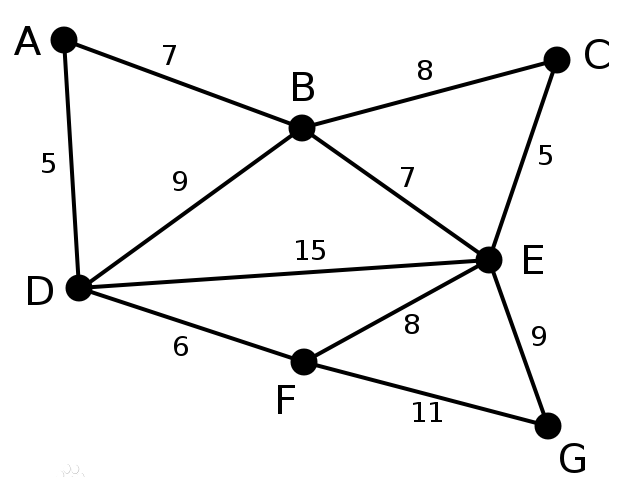2、顶点 D D 被任意选为起始点。顶点 A 、 B 、 E A、B、E F F 通过单条边与 D D 相连。 A A 是距离 D D 最近的顶点，因此将 A A 及对应边 A D AD 以高亮表示。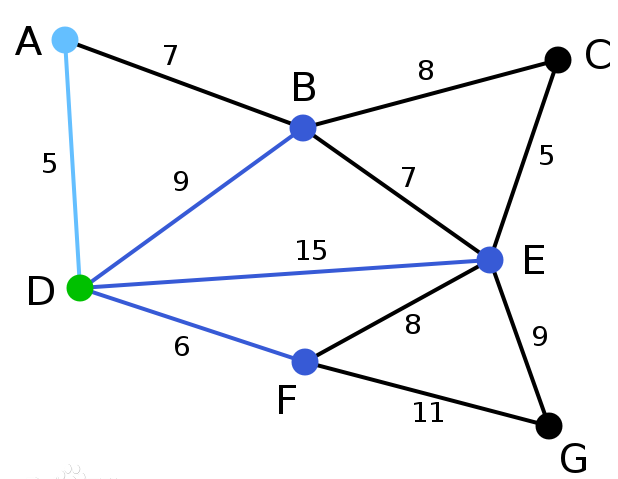3、下一个顶点为距离 D 或 A D或A 最近的顶点。 B 距 D 为 9 ， 距 A 为 7 ， E 为 15 ， F 为 6 B距D为9，距A为7，E为15，F为6 。因此， F 距 D 或 A F距D或A 最近，因此将顶点 F 与 相 应 边 D F F与相应边DF 以高亮表示。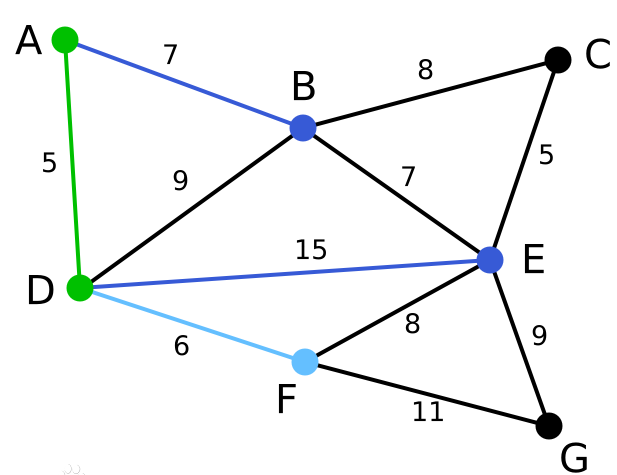4、 算 法 继 续 重 复 上 面 的 步 骤 。 距 离 A 为 7 的 顶 点 B 被 高 亮 表 示 算法继续重复上面的步骤。距离A为7的顶点B被高亮表示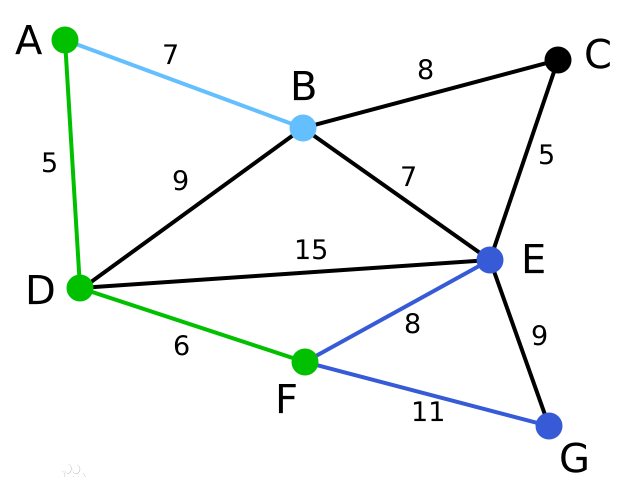5、在当前情况下，可以在 C 、 E 与 G C、E与G 间进行选择。 C 距 B 为 8 ， E 距 B 为 7 ， G 距 F 为 11 C距B为8，E距B为7，G距F为11 。点 E E 最近，因此将顶点 E E 与相应边 B E BE 高亮表示。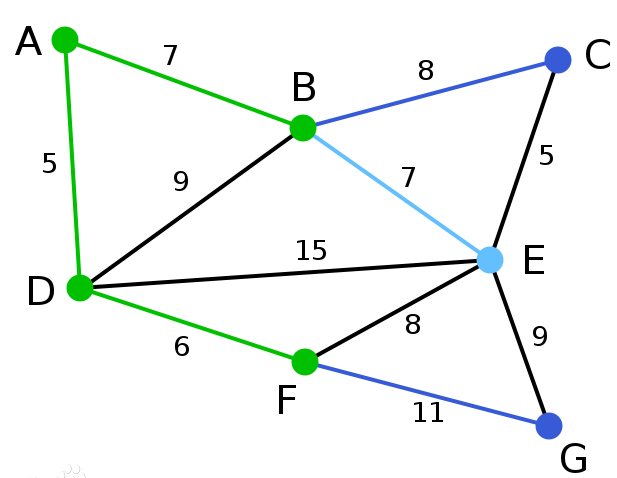6、这里，可供选择的顶点只有 C 和 G 。 C 距 E 为 5 ， G 距 E 为 9 ， 故 选 取 C ， 并 与 边 E C C和G。C距E为5，G距E为9，故选取C，并与边EC 一同高亮表示。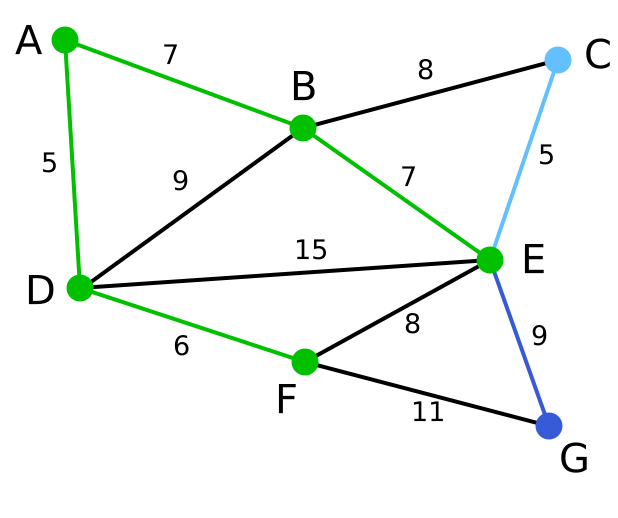7、顶点 G G 是唯一剩下的顶点，它距 F F 11 11 距 E 为 9 ， E 最 近 ， 故 高 亮 表 示 G 及 相 应 边 E G 距E为9，E最近，故高亮表示G及相应边EG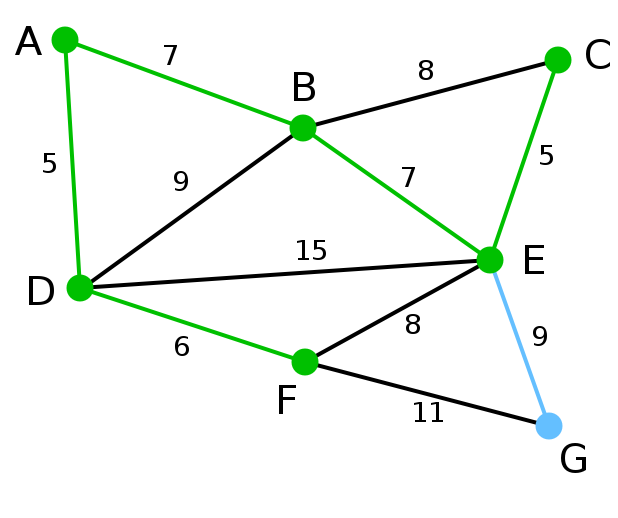8、所有顶点均已被选取，图中绿色部分即为连通图的最小生成树。在此例中，最小生成树的权值之和为 39 39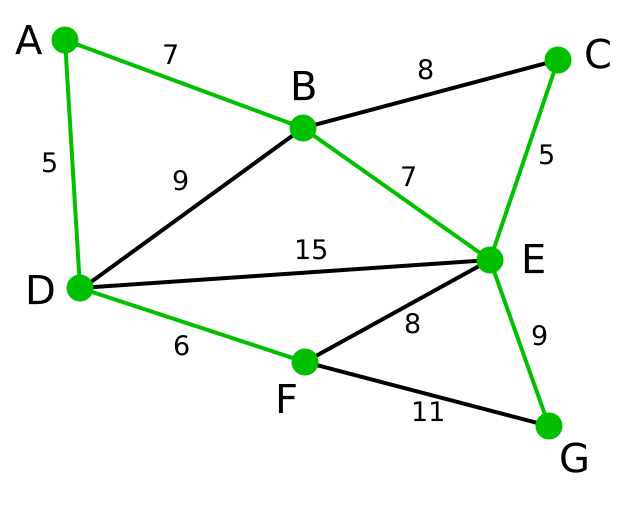代码分析

把图存入 G r a p h [ N ] [ N ] Graph[N][N]

int Graph[N][N]={
{M, M, M, M, M, M, M},
{M, 0, 6, 1, 5, M, M},
{M, 6, 0, 5, 3, M, M},
{M, 1, 5, 0, 5, 6, 4},
{M, 5, 3, 5, 0, M, 2},
{M, M, M, 6, M, 0, 6},
{M, M, M, 4, 2, 6, 0},
};

调用 P r i m Prim 方法

int prim(int graph[][N])
{
int n = 6;
int lowcost[M];//记录边对应的权值
int mst[M];//记录权值对应的边  起点是值  终点是下标
int i, j, min, minid, sum = 0;
for (i = 2; i <= n; i++)
{
lowcost[i] = graph[i];
mst[i] = 1;
}
mst = 0;
for (i = 2; i <= n; i++)
{
min = M;
minid = 0;
for (j = 2; j <= n; j++)
{
if (lowcost[j] < min && lowcost[j] != 0)
{
min = lowcost[j];
minid = j;
}
}
cout << "V" << mst[minid] << "-V" << minid << "=" << min << endl;
sum += min;
lowcost[minid] = 0;
for (j = 2; j <= n; j++)
{
if (graph[minid][j] < lowcost[j])
{
lowcost[j] = graph[minid][j];
mst[j] = minid;
}
}
}
return sum;
}

P r i m Prim 方法中第一个 f o r for 循环把第一个点对应的边(权值)放入 l o w c o s t [ i ] lowcost[i] 数组中
m s t [ ] mst[] 数组，其下标记录终点，下标对应的值是起点。这样就记录了一条边

主要做一个初始化的工作

int lowcost[M];//记录边对应的权值
int mst[M];//记录权值对应的边  起点是值  终点是下标
int i, j, min, minid, sum = 0;
for (i = 2; i <= n; i++)
{
lowcost[i] = graph[i];
mst[i] = 1;
}
mst = 0;

第二个 f o r for 循环，每一次都能找到一条最小的边

for (i = 2; i <= n; i++)
{
min = M;
minid = 0;
for (j = 2; j <= n; j++)
{
if (lowcost[j] < min && lowcost[j] != 0)
{
min = lowcost[j];
minid = j;
}
}
cout << "V" << mst[minid] << "-V" << minid << "=" << min << endl;
sum += min;
lowcost[minid] = 0;
for (j = 2; j <= n; j++)
{
if (graph[minid][j] < lowcost[j])
{
lowcost[j] = graph[minid][j];
mst[j] = minid;
}
}
}

第二个 f o r for 循环中的第一个 f o r for 循环会找到当前边集中权值最小的，并且记录其权值和下标.

min = M;
minid = 0;
for (j = 2; j <= n; j++)
{
if (lowcost[j] < min && lowcost[j] != 0)
{
min = lowcost[j];
minid = j;
}
}
cout << "V" << mst[minid] << "-V" << minid << "=" << min << endl;
sum += min;
lowcost[minid] = 0;

第二个 f o r for 循环中的第二个 f o r for 循环会找到当前新加入的边中权值比已经存入的更小的，并且记录其权值和下标.

for (j = 2; j <= n; j++)
{
if (graph[minid][j] < lowcost[j])
{
lowcost[j] = graph[minid][j];
mst[j] = minid;
}
}

代码

#include<iostream>
using  namespace std;

#define M 65535 //无穷大
#define N 8  //顶点数

int prim(int graph[][N])
{
int n = 6;
int lowcost[M];//记录边对应的权值
int mst[M];//记录权值对应的边  起点是值  终点是下标
int i, j, min, minid, sum = 0;
for (i = 2; i <= n; i++)
{
lowcost[i] = graph[i];
mst[i] = 1;
}
mst = 0;
for (i = 2; i <= n; i++)
{
min = M;
minid = 0;
for (j = 2; j <= n; j++)
{
if (lowcost[j] < min && lowcost[j] != 0)
{
min = lowcost[j];
minid = j;
}
}
cout << "V" << mst[minid] << "-V" << minid << "=" << min << endl;
sum += min;
lowcost[minid] = 0;
for (j = 2; j <= n; j++)
{
if (graph[minid][j] < lowcost[j])
{
lowcost[j] = graph[minid][j];
mst[j] = minid;
}
}
}
return sum;
}

int main()
{
int cost;
int Graph[N][N]={
{M, M, M, M, M, M, M},
{M, 0, 6, 1, 5, M, M},
{M, 6, 0, 5, 3, M, M},
{M, 1, 5, 0, 5, 6, 4},
{M, 5, 3, 5, 0, M, 2},
{M, M, M, 6, M, 0, 6},
{M, M, M, 4, 2, 6, 0},
};
//求解最小生成树
cost = prim(Graph);
//输出最小权值和
cout << "最小权值和=" << cost << endl;
system("pause");
return 0;
}

展开全文算法 c语言 数据结构
• int Prim(int graph[MAX][MAX], int n){ /* lowcost[i]记录以i为终点的边的最小权值，当lowcost[i]=0时表示终点i加入生成树 */ int lowcost[MAX]; /* mst[i]记录对应lowcost[i]的起点 */ int mst[MAX]; int i, ...
• Prim算法的大致思想：假设图G顶点集合为U，首先任选一点a(默认为第一条边)作为起始点，加入集合V,再从集合U-V中找到另一点b使得b到V中任意一点的权值最小，把b加入集合V。以此类推，现在集合V={a，b}，再从集合U-V...

算法描述：

Prim算法的大致思想：假设图G顶点集合为U，首先任选一点a(默认为第一条边)作为起始点，加入集合V,再从集合U-V中找到另一点b使得b到V中任意一点的权值最小，把b加入集合V。以此类推，现在集合V={a，b}，再从集合U-V找到一点c使得c到V中任意一点的权值最小，将c加入集合V，此时就构建出一棵最小生成树。

为了便于在集合U和U-V之间选择权值最小的边，建立两个数组mst和lowcost。

lowcost[i]：表示以i为终点的边的最小权值，当lowcost[i]=0说明i点加入了最小生成树。

mst[i]:表示对应lowcost[i]的起点，即说明边（mst[i],i）是最小生成树的一条边，当mst[i]=0时说明起点i加入了最小生成树。

代码实现

#include <stdio.h>

#define MAX 100
#define INF 0x3f3f3f3f

int Prim(int graph[][MAX], int n)
{
int lowcost[MAX], mst[MAX];
/*
lowcost[i]记录以i为终点的边的最小权值，当lowcost[i]=0时表示终点i加入生成树
mst[i]记录对应lowcost[i]的起点，当mst[i]=0时表示起点i加入生成树
*/
int i, j, min, minid, sum = 0;

for (i = 2; i <= n; i++)  //默认选择1号节点加入生成树，从2号节点开始初始化
{
lowcost[i] = graph[i];  //最短距离初始化为其他节点到1号节点的距离
mst[i] = 1;  //标记所有节点的起点皆为默认的1号节点
}

mst = 0;  //标记1号节点加入生成树

for (i = 2; i <= n; i++)  //n个节点至少需要n-1条边构成最小生成树
{
min = INF;
minid = 0;

for (j = 2; j <= n; j++)  //找满足条件的最小权值边的节点minid
{
if (lowcost[j] < min && lowcost[j] != 0)  //边权值较小且不在生成树中
{
min = lowcost[j];
minid = j;
}
}
//数字转换为字符
printf("%c - %c : %d\n", mst[minid] + 'A' - 1, minid + 'A' - 1, min);  //输出生成树边的信息:起点，终点，权值

sum += min;  //累加权值
lowcost[minid] = 0;  //标记节点minid加入生成树

for (j = 2; j <= n; j++)  //更新当前节点minid到其他节点的权值
{
if (graph[minid][j] < lowcost[j])  ///发现更小的权值
{
lowcost[j] = graph[minid][j];  //更新权值信息
mst[j] = minid;  //更新最小权值边的起点
}
}
}
return sum;  //返回最小权值和
}
void gen(int graph[MAX][MAX],int vertex,int edge)
{
char chx, chy;
int  weight;
getchar();
for (int i = 1; i <= vertex; i++)  //初始化图，所有节点间距离为无穷大
for (int j = 1; j <= vertex; j++)
graph[i][j] = INF;
for (int k = 0; k < edge; k++)  //读取边信息
{
scanf("%c %c %d", &chx, &chy, &weight);
getchar();
int i = chx - 'A' + 1, j = chy - 'A' + 1;  ///ABCDEF
graph[i][j] = weight;//无向图
graph[j][i] = weight;
}
}

int main()
{
int m, n;

int graph[MAX][MAX];

scanf("%d %d", &m, &n);  //读取节点和边的数目
gen(graph,m,n);
printf("Total: %d\n", Prim(graph, m));

return 0;
}

输入：

6 10
A B 6
A C 1
A D 5
B C 5
B E 3
C E 6
C F 4
C D 4
E F 6
D F 2

输出：

A - C : 1
C - D : 4
D - F : 2
C - B : 5
B - E : 3
Total: 15

展开全文• Prim算法C语言实现

千次阅读 2016-06-02 00:47:49
void Prim(MGraph *mgraph, int v); int main(void ) { MGraph *mgraph; mgraph=build_graph(); Prim(mgraph, 0); return 0; } MGraph *build_graph() { MGraph *mgraph; int i,j; int this_edges=0; int...
#include <stdio.h>
#include <stdlib.h>
#include <limits.h>
#define NUM 6

typedef struct Node
{
int num;
int data;
} Node;
typedef struct MGraph
{
int edges[NUM][NUM];
int n,e;
} MGraph;

MGraph *build_graph();
void Prim(MGraph *mgraph, int v);

int main(void )
{
MGraph *mgraph;

mgraph=build_graph();
Prim(mgraph, 0);
return 0;
}

MGraph *build_graph()
{
MGraph *mgraph;
int i,j;
int this_edges=0;
int arrays[NUM][NUM]={{0,6,1,5,INT_MAX,INT_MAX},
{6,0,5,INT_MAX,3,INT_MAX},
{1,5,0,5,6,4},
{5,INT_MAX,5,0,INT_MAX,2},
{INT_MAX,3,6,INT_MAX,0,6},
{INT_MAX,INT_MAX,4,2,6,0}};
mgraph=(MGraph *)malloc(sizeof(MGraph));
for(i=0;i<NUM;i++)
{
for(j=0;j<NUM;j++)
{
mgraph->edges[i][j]=arrays[i][j];
if(arrays[i][j]!=0 && arrays[i][j]!=INT_MAX)
{
this_edges++;
}
}
}
mgraph->n=NUM;
mgraph->e=this_edges;

printf("node=%d,edges=%d\n",mgraph->n,mgraph->e);
for(i=0;i<NUM;i++)
{
for(j=0;j<NUM;j++)
{
if(mgraph->edges[i][j]!=INT_MAX)
{
printf("%3d",mgraph->edges[i][j]);
}
else
{
printf("%3c",'&');
}
}
printf("\n");
}

return mgraph;

}
void Prim(MGraph *mgraph, int v)
{
int i,j;
int min,index;
int dis[NUM];
int pre[NUM];

for(i=0;i<mgraph->n;i++)
{
dis[i]=mgraph->edges[v][i];
pre[i]=v;
}

for(j=1;j<mgraph->n;j++)
{
min=INT_MAX;
index=v;
for(i=0;i<mgraph->n;i++)
{
if(dis[i]!=0 && dis[i]!=INT_MAX)
{
if(dis[i]<min)
{
min=dis[i];
index=i;
}
}
}
printf("%d-%d:%d\n",pre[index],index,min);

for(i=0;i<mgraph->n;i++)
{
if(mgraph->edges[i][index]<dis[i])
{
dis[i]=mgraph->edges[i][index];
pre[i]=index;
}
}
}
}

展开全文Prim算法
•prim
• 功能模块：Prim算法实现最小生成树 适用领域：无向图 基础模块：Graph 辅助数组 */ #include<stdio.h> #include<stdlib.h> #define MAXSIZE 20 typedef char VertexType; typedef s

问题

使用Prim算法，进行Minimum spanning tree，从点A开始

示例图7 12
A
B
C
D
E
F
G
AB 2
AC 4
BD 3
CD 2
BE 10
DE 7
CF 5
DF 8
FG 1
DG 4
EG 6
A

代码

/*
功能模块：Prim算法实现最小生成树
适用领域：无向图
基础模块：Graph 辅助数组
*/

#include<stdio.h>
#
展开全文c语言 数据结构 算法
• Prim ( Graph * graph , int n , int dist [ MAXSIZE ] , int parent [ MAXSIZE ] ) { //dist=1; parent [ 0 ] = - 1 ; int vcount = 0 ; while ( 1 ) { int min = 11112 ; int v = - 1 ; ...
• 输入数据: 7 11 A B 7 A D 5 B C 8 B D 9 B E 7 C E 5 D E 15 D F 6 E F 8 E G 9 F G 11 输出: A - D : 5 D - F : 6 A - B : 7 B - E : 7 E - C : 5 E - G : 9 Total:39prim
• 算法介绍： Prim算法是计算带权连通图最小生成树的经典算法，与计算单源最短路径的Dijkstra算法相似，Prim算法同属于贪心算法，即在求解时每次都做出最优解，zuiprim 数据结构 c语言 算法
• 离散大作业 最小生成树算法 一Prim算法 设G=(V,E)是连通带权图V={1,2,n}构造G的最小生成树的Prim算法的基本思想是 (1)置S={1} (2)只要S是V的真子集就作如下的贪心选择 选取满足条件i Sj V-S且c[i][j]最小的边将顶点j...
• 普里姆算法介绍普里姆(Prim)算法，和克鲁斯卡尔算法一样，是用来求加权连通图的最小生成树的算法。基本思想对于图G而言，V是所有顶点的集合；现在，设置两个新的集合U和T，其中U用于存放G的最小生成树中的顶点，T...prim算法c语言
• 最小生成树——Prim算法（C语言实现）

千次阅读 多人点赞 2020-11-21 15:52:40
最小生成树（Prim算法） 应用领域 基本概念 1）生成树：连通图的生成树是包含全部顶点的极小连通子图。（含有n-1条边）（从任意节点出发都能到达任意一个顶点） 2）生成树代价：在无向连通网中，生成树上各边的...
• C语言实现Prim算法

千次阅读 2021-10-24 21:19:26
C语言实现Prim算法话不多说，思想简单，直接上代码 维护两个数组。 一个lowcost数组，表示当前已经归入最小生成树的结点集合与未归入的集合节点之间的最小代价； 一个visited数组，表示是否已经归入最小生成树 每次...
• Prim（普利姆）算法c语言实现

千次阅读 多人点赞 2020-05-08 13:39:24
关于最小生成树的算法，那么肯定要提到大名鼎鼎的prim和kruskal算法， 这两种算法思想大同小异。对于算法的学习，我的经验是搞懂思想，分 模块去记忆，而不是去背代码，虽然短时间内，...##Prim算法：（ 三步走） ...算法 数据结构 c语言
• 砍掉一条则不连通，增加一条边则会出现回路 （二）Prim 算法（普里姆） Prim 算法（普里姆）：从某一个顶点开始构建生成树；每次将代价最小的新顶点纳入生成树，直到所有顶点都纳入为止。 从P城开始进行建成生成树。...最小生成树
• Prim算法的C语言程序

千次阅读 2017-02-04 22:53:12
Prim算法是有关图的最小生成树的算法。1957年由美国计算机科学家罗伯特·普里姆（Robert C. Prim）独立发现。 程序来源：Prim's Algorithm。 百度百科：Prim算法。 维基百科：Prim's Algorithm。 需要说明的是，...prim算法
• /* 求最小生成树的prim算法 */#include #include #define MaxSize 20#define MAX 10000typedef char VertexType;//定义图 的邻接矩阵表示法结构typedef struct Graph {VertexType ver[MaxSize+1];int edg[MaxSize]...prim算法c语言
• prim算法就好像是一棵"生成树"在慢慢长大，从开始的一个顶点长到了n个顶点。 总结一下这个算法，将图中所有的顶点分为2类，树顶点（已被选入生成树的顶点）和非树顶点（还未被选入生成树的顶点），接下来要找出一条...
• 最小生成树Prim算法朴素版 C语言实现

万次阅读 多人点赞 2017-11-25 11:03:25c语言 算法 数据结构 prim 最小生成树
•prim算法
• Prim算法通过不断地增加生成树的顶点来得到最小生成树。在算法的任一时刻，一部分顶点已经添加到生成树的顶点集合中，而其余顶点尚未加到生成树中。此时，Prim算法通过选择边，使得的权值是所有u(起点)在生成树中，...
• 下面是编程之家 jb51.cc 通过网络收集整理的代码片段。编程之家小编现在分享给大家，也给大家做个参考。#include #include #define STACK_INIT_SIZE 20#define STACKINCREMENT 10typedef char ElemType;...
• Prim算法 算法实现 算法证明 Kruskal算法 算法实现 算法证明 最小生成树简介 最小生成树（MST）：给定一加权无向图，找出它的一颗最小生成树。 定义：图的最小生成树是它的一副含有其所有顶点的...c 算法
• // Prim算法实现(采用邻接表存储).cpp : Defines the entry point for the console application.//#include "stdafx.h"#include "stdafx.h"#include#define MAX 100#define Infinity 65535typedef int WeiType;......

prim算法c语言c语言 订阅